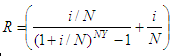Bank Withdrawal - regular withdrawals from an investment

This article develops a script in Matlab to calculate bank withdrawals, i.e., the maximum amount which may be withdrawn regularly from an investment over a specified time period.

All withdrawals are assumed to be equal. You must provide the amount of the initial investment, the nominal interest rate, the number or withdrawals per year and the number of years.

The maximum amount of withdrawals is calculated by the following formulawhere:
R = amount of regular withdrawal
P = initial investment
i = nominal interest rate
N = number of withdrawals per year
Y = number or years

Because this program calculates a maximum amount, a balance of \$0.00 will be left in your account at the end of the time period. You may withdraw any lesser amount under the same conditions and leave a remaining balance in your account.

This is our Matlab function or script to calculate the above formula:

function r = bank_withdrawal(p, it, n, y)
it = it/(n*100);

r = p*(it/((1 + it)^(n*y) - 1) + it);

We can create another script to test and run the above m-file:

clc; clear; format bank

p = input('Enter initial investment: ');
it = input(
'Enter nominal interest rate: ');
n = input(
'Enter number of withdrawals per year: ');
y = input(
'Enter number of years: ');

amount_of_bank_withdrawal = bank_withdrawal(p, it, n, y)

Example:

Joe invests \$8000 at 9.5%. He plans to make regular withdrawals every month for ten years, leaving nothing at the end. Ho much should he withdraw each time?

We run our test code and get:

Enter initial investment: 8000
Enter nominal interest rate: 9.5
Enter number of withdrawals per year: 12
Enter number of years: 10

The result is:

amount_of_bank_withdrawal = 103.52

From 'Bank Withdrawal' to home

From 'Bank Withdrawal' to 'Finance Formulas'

 Top Initial investments Nominal interest rate Effective interest rate Calculate annuity